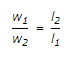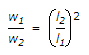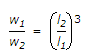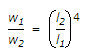# Online Civil Engineering Test - RCC Structures Design TestLoading Test...

Instruction:

• This is a FREE online test. DO NOT pay money to anyone to attend this test.
• Total number of questions : 20.
• Time alloted : 30 minutes.
• Each question carry 1 mark, no negative marks.
• DO NOT refresh the page.
• All the best :-).

1.

As per I.S. 456 - 1978, the pH value of water shall be

A.
 less than 6B.
 equal to 6C.
 not less than 6D.
 equal to 72.

The design of a retaining wall assumes that the retained earth

A.
 is dryB.
 is free from moistureC.
 is not cohesivesD.
 consists of granular particlesE.
 all the above.3.

Dimensions of a beam need be changed if the shear stress is more than

A.
 10 kg/cm2B.
 15 kg/cm2C.
 20 kg/cm2D.
 25 kg/cm24.

A reinforced concrete cantilever beam is 3.6 m long, 25 cm wide and has its lever arm 40 cm. It carries a load of 1200 kg at its free end and vertical stirrups can carry 1800 kg. Assuming concrete to carry one-third of the diagonal tension and ignoring the weight of the beam, the number of shear stirrups required, is

A.
 30B.
 35C.
 40D.
 45E.
 505.

Pick up the true statement from the following:

A.
 Plain ceiling provides the best property diffusing lightB.
 In the absence of beams, it is easier to install pipingC.
 In the absence of beams, it is easier to paintD.
 A flat slab is capable to withstand concentrated loadsE.
 All the above.6.

In a singly reinforced beam, the effective depth is measured from its compression edge to

A.
 tensile edgeB.
 tensile reinforcementC.
 neutral axis of the beamD.
 longitudinal central axis.7.

Pick up the incorrect statement from the following: Tensile reinforcement bars of a rectangular beam

A.
 are curtailed if not required to resist the bending momentB.
 are bent up at suitable places to serve as shear reinforcementC.
 are bent down at suitable places to serve as shear reinforcementD.
 are maintained at bottom to provide at least local bond stress.8.

A part of the slab may be considered as the flange of the T-beam if

A.
 flange has adequate reinforcement transverse to beamB.
 it is built integrally with the beamC.
 it is effectively bonded together with the beamD.
 all the above.9.

A.
 semi-hemisphereB.
 ellipsoidC.
 parabolloidD.
 none of these.10.

An R.C.C beam of 25 cm width has a clear span of 5 metres and carries a U.D.L. of 2000 kg/m inclusive of its self weight. If the lever arm of the section is 45 cm., the beam is

A.
 safe in shearB.
 is safe with stirrupsC.
 is safe with stirrups and inclined membersD.
 needs revision of the section.11.

After prestressing process is completed, a loss of stress is due to

A.
 shrinkage of concreteB.
 elastic shortening of concreteC.
 creep of concreteD.
 creep of steelE.
 all the above.12.

In case the factor of safety against sliding is less than 1.5, a portion of slab is constructed downwards at the end of the heel slab, which is known as

A.
 a keyB.
 a cut-off wallC.
 a ribD.
 all the above.13.

If the length of an intermediate span of a continuous slab is 5m, the length of the end span is kept

A.
 4.5 mB.
 4.0 mC.
 3.5 mD.
 3.0 mE.
 none of these.14.

Spacing of stirrups in a rectangular beam, is

A.
 kept constant throughout the lengthB.
 decreased towards the centre of the beamC.
 increased at the endsD.
 increased at the centre of the beam.15.

Minimum spacing between horizontal parallel reinforcement of the same size should not be less than

A.
 one diameterB.
 2.5 diametersC.
 3 diametersD.
 3.5 diametersE.
 4 diameters16.

Side face reinforcement shall be provided in the beam when depth of the web in a beam exceeds

A.
 50 cmB.
 75 cmC.
 100 cmD.
 120 cm17.

Enlarged head of a supporting column of a flat slabs is technically .known as

A.
 supporting end of the columnB.
 top of the columnC.
 capitalD.
 drop panelE.
 none of these.18.

The diameter of main bars in R.C.C. columns, shall not be less than

A.
 6 mmB.
 8 mmC.
 10 mmD.
 12 mm19.

The minimum thickness of the cover at the end of a reinforcing bar should not be less than twice the diameter of the bar subject to a minimum of

A.
 10 mmB.
 15 mmC.
 20 mmD.
 25 mmE.
 30 mm20.

If l1 and l2 are the lengths of long and short spans of a two way slab simply supported on four edges and carrying a load w per unit area, the ratio of the loads split into w1 and w2 acting on strips parallel to l2 and l1 is

A.B.C.D.